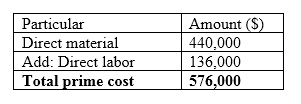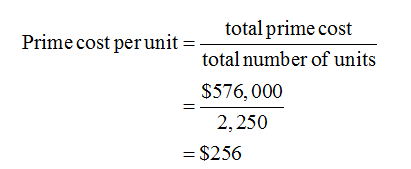# Prime Cost and Conversion CostGrin Company manufactures digital cameras. In January, Grin produced 2,250 cameras with the following costs:Direct materials\$440,000Direct labor136,000Manufacturing overhead736,000There were no beginning or ending inventories of WIP.Required:If required, round your answers to the nearest cent.1.  What was the total prime cost in January?\$2.  What was the prime cost per unit in January?\$per unit3.  What was the total conversion cost in January?\$4.  What was the conversion cost per unit in January?\$per unit

Question
13 views

Prime Cost and Conversion Cost

Grin Company manufactures digital cameras. In January, Grin produced 2,250 cameras with the following costs:

 Direct materials \$440,000 Direct labor 136,000 Manufacturing overhead 736,000

There were no beginning or ending inventories of WIP.

Required:

1.  What was the total prime cost in January?
\$

2.  What was the prime cost per unit in January?
\$per unit

3.  What was the total conversion cost in January?
\$

4.  What was the conversion cost per unit in January?
\$per unit

check_circle

Step 1

The prime cost is the cost of direct labor and direct material used. It is calculated by adding the cost of direct material used and direct labor.

The conversion cost is referred to the cost incurred in converting the raw material into the finished goods. It is calculated by adding the cost of manufacturing overhead in the cost of direct labor.

Step 2

1)
Total prime cost in January is calculated as follows:Step 3

2)

Prime cost per unit in January:

Total prime cost: \$576,000

Total number of units: 2,250...

### Want to see the full answer?

See Solution

#### Want to see this answer and more?

Solutions are written by subject experts who are available 24/7. Questions are typically answered within 1 hour.*

See Solution
*Response times may vary by subject and question.
Tagged in